# Is pH the Measurement of Hydrogen Ion Concentration or Ion Activity?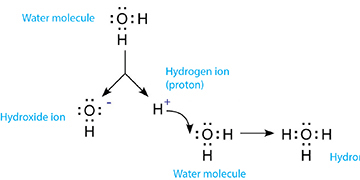## What Does a pH Meter Measure?

YSI has established a reputation as experts in dissolved oxygen measurement, but our extensive experience in the field and lab demonstrates our proficiency in the measurement of a multitude of parameters including the measurement of pH.

What does pH measure? Does ph measure hydronium ion concentration?

pH is one of the most fundamental parameters that is measured in nearly every application and we will answer these questions. Due to its importance, we need to review fundamental topics such as the dissociation of water and the hydrogen ion, the pH scale, and the anatomy of the glass membrane electrode.

We are focused on providing resources to help ensure you get accurate pH measurements and have developed a practical guide to pH, the pH Handbook

To begin our discussion of pH, let’s review and establish exactly what we are measuring.

### Dissociation of Water and Formation of the Hydronium Ion

Whether an aqueous solution reacts as an acid or a base depends on its hydrogen ion (H+) content. Even chemically pure, neutral water contains hydrogen ions due to the auto dissociation of water. In this process, water molecules are broken up into simpler constituents (i.e. ions). The dissociation of water is represented in equation :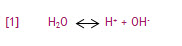In this reaction, H2O is deprotonated (i.e. loses a proton). This results in the formation of a positively charged hydrogen ion (H+) and a negatively charged hydroxide ion (OH-). The hydrogen ion is customarily used to represent a proton.

The hydrogen ion does not remain a free proton for long, as it is quickly hydrated by a surrounding un-ionized water molecule. The formation of the resulting ion, the hydronium ion, is represented in equation :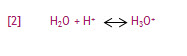Figure 1 combines equations  and  to show the sequence of hydronium ion formation.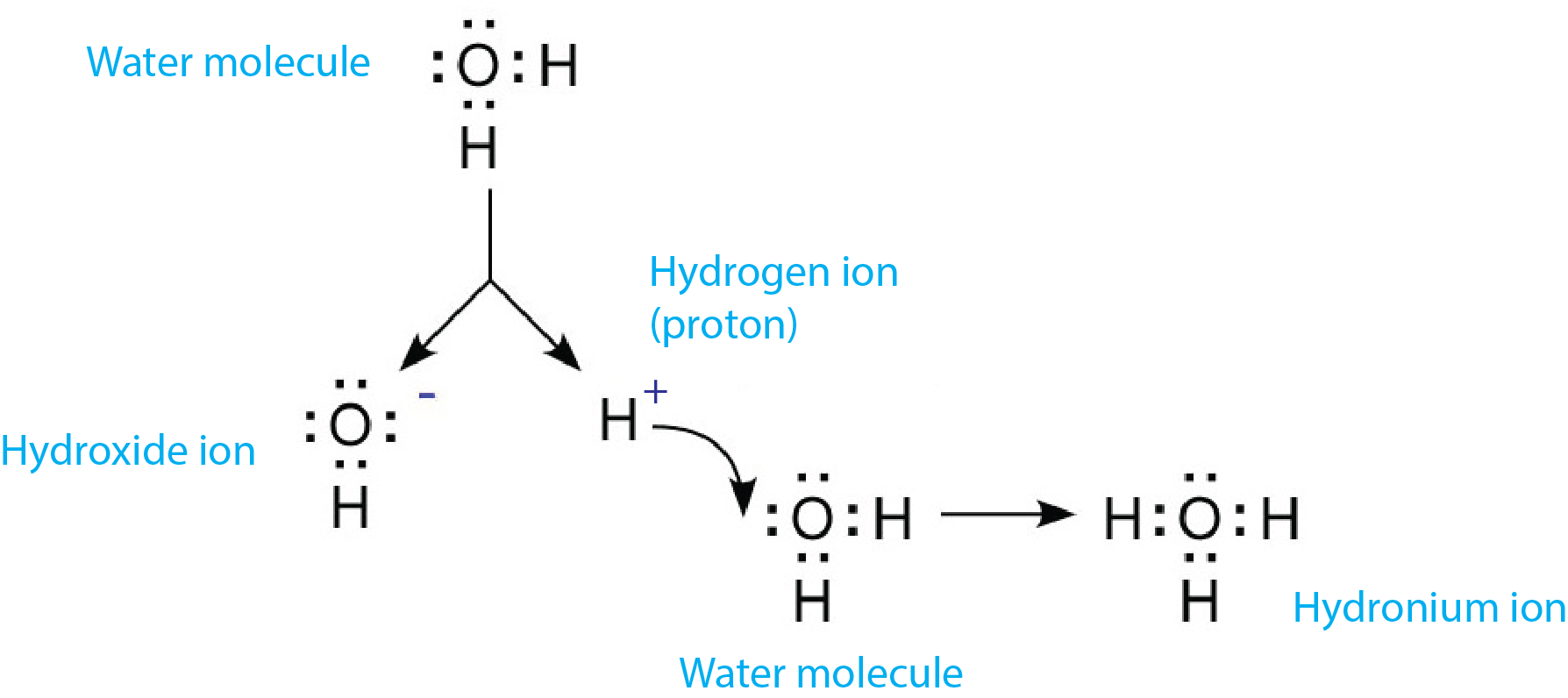Figure 1: Dissociation of water and the formation of the hydronium ion

At equilibrium conditions (750 mmHg and 25°C), 1 L of pure, neutral water contains 10-7 mol H+ and 10-7 mol OH- ions.

### Is It An Acid or A Base?

Acids are substances that release hydrogen ions (i.e. protons), so a solution is considered acidic if it contains more hydrogen ions than neutral water.

Bases are substances that accept hydrogen ions. When bases are dissolved in water, they bind to some of the hydrogen ions formed from the dissociation of the water. Basic solutions contain fewer hydrogen ions than neutral water.

Therefore, aqueous solutions are considered acidic if they contain more than 10-7 mol/L of hydrogen ions and basic if they contain fewer than 10-7mol/L of hydrogen ions at 25°C.

Acids and bases neutralize each other, resulting in the formation of water and salt. An example would be the reaction of sodium hydroxide (NaOH) and hydrochloric acid (HCl) in equation :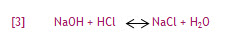A reaction between an acid and a base involves a transfer of protons. In the above acid-base reaction, a proton is donated from HCl (acid) to NaOH (base) to form sodium chloride (NaCl) and water.

### Ion Activity and Ion Concentration

Ions carry either a positive (e.g. H+) or negative (e.g. OH-) charge.  As charge carriers, all dissolved ions exert electrical forces on their surroundings. While a solution might be electrically neutral on a macroscopic scale, the effects of ions can be drastic at the microscopic scale.

Solutions with a relatively high concentration of ions may yield a determination of ion concentration that is unusually low. Therefore, solutions begin to behave as if some of the ions are no longer present. This apparent loss of ions is caused by the interaction of ions in solution, ultimately resulting in significant deviations from ideal behavior. In order to take this interaction into account, the ion activity, also known as the effective ion concentration, must be considered rather than ion concentration. As a result, pH is a measure of hydrogen ion activity.

Hydrogen ion activity can be determined by equation :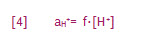aH+= hydrogen ion activity

f =hydrogen ion activity coefficient

[H+] = hydrogen ion concentration

Example:

If the activity coefficient is determined to be 0.91 and the hydrogen ion concentration is 100 mol/kg*, the ion activity would be equal to 91

*Although ion activity and the activity coefficient have no units, their magnitude depends on the concentration units used

The activity coefficient is a function of the ion concentration and approaches 1 as the solution becomes increasingly dilute. Therefore, hydrogen ion concentration and hydrogen ion activity are nearly equal in very dilute samples. In samples with high ion concentration, ion activity becomes much lower than the concentration (i.e. apparent loss of ions).

YSI offers a variety of platforms for the measurement of pH. Whether for the lab (MultiLab, TruLab), environmental sampling (Pro Quatro, ProDSS, Pro10), or for long-term monitoring (EXO1, EXO2), YSI has what you need!

What does pH Measure? pH is the measurement of hydrogen ion activity.

# Additional Blog Posts of Interest:

Why is the pH Scale Logarithmic?

Extend the Life of Your pH Electrode in 3 Practical Steps

pH Meter Calibration Problems? Check out These 12 Tips!

4 Steps to GLP Compliant pH Measurement and Performance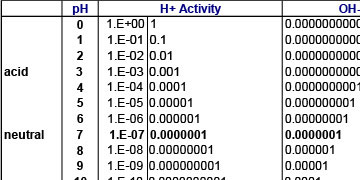Why is the pH Scale Logarithmic?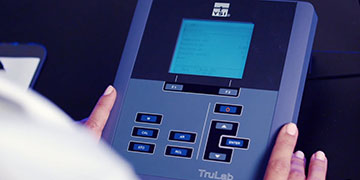Lab Instruments and Electrodes for pH, ORP and ISEs

More a question than a comment Is the composition of say a commercial pH 4.0 buffer solution adjusted such that its hydrogen ion concentration is 10^-4 M is it that its hydrogen ion activity is 10^-4 M in which case presumably one has to estimate its activity coefficient from its composition (Davies equation or other) in order to determine the hydrogen ion concentration corresponding to the pH 4 value

The short answer is that all ISE’s, including a pH probe, are measuring activity. For most applications, we can consider the activity to be equal to the concentration in a pH probe; this is less true for other ISEs that experience interferences more readily from more different sources. Probably the most infamous instances of the activity not being equal to the concentration is the “Alkaline Error” (sometimes called the “Sodium Error”). At pH values of 12 or above (approximately), Na+ and other ions can start to interfere with the silica layer on the outside of the pH bulb. The higher the pH, the worse this interference gets, but only once we have already surpassed pH 12 or so; even the alkaline error at pH14 is still closer to pH14 than pH 13 or 15, for example. The degree of this error is dependent upon the construction of the probe. In the example of the pH4 buffer, the concentration and the activity are going to be so similar that the difference between them is negligible. The intrinsic error of the pH probe will, in practically all cases, be larger than any correction that could be performed by having the probe look for “true concentration” instead of the activity. Again, for a large majority of pH readings, the activity is practically equal to the concentration. Great question!